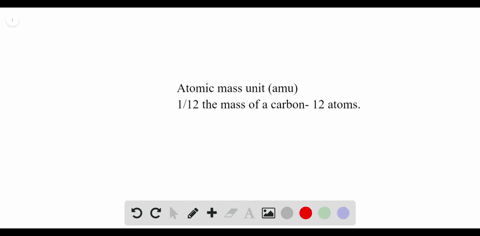### Standard Units Define the atomic mass unit. What …

00:21University of Miami
Problem 62

What do the superscript and subscript in the notation
$\stackrel{40}{19} \mathrm{K}$ represent?

The superscript $(40)$ indicate the mass number, while the subscript $(19)$ indicate the atomic number.

## Discussion

You must be signed in to discuss.

## Video Transcript

I will work on problem 62 from chapter four. This problem were asked about some notation or an isotope. And what did the superscript and subscript represent in the example that were given here is for TASI, um, with 40 and 19. So the superscript 40 represents Ah, mess number and the's sub script 19 represents atomic number. So another these air the terms but mass number means a number of neutrons, uh, neutrons, plus protons, whereas atomic number is just the number of protons.